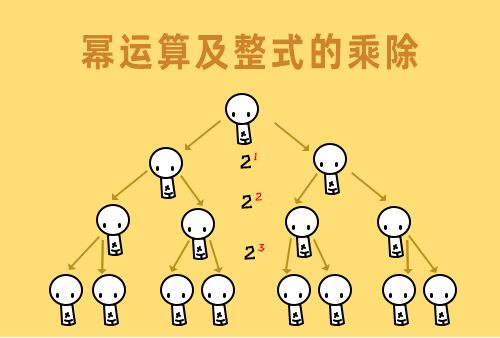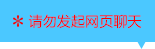| | |

||
APP端下载AndroidiPhone
￥198

|
1964人点赞
116605人已学习
|

3天无理由退款

3天无理由退款：退款将以超级币形式退至您的超级课堂学习账户，便于您重新选购其他课程。恶意退款将被冻结账号。

• 1、二次函数的一般式：$y=ax^{2}+bx+c$（$a$、$b$、$c$为常数，且$a\neq 0$）。
2、 判定二次函数的依据：自变量的最高次是二次，且二次项系数不为零，且解析式的右边一定是整式，不能包含分式或根式
3、 二次函数解析式的求法，还是待定系数法，一般有几个未知系数就要代入几组$xy$值，其实就是解多元方程
• 1、二次函数$y=ax^{2}$（$a\neq 0$）的图象是抛物线，顶点就是原点，对称轴是$y$轴
2、 $a$的正负决定了开口方向，$a> 3、 0$时，开口向上，$a< 4、 0$时，开口向下
5、 增减性的判断，根据$a$的符号画出大致图像，然后判断$y$随$x$变化而变化的趋势，也就是增减性
6、 $\left | a \right |$决定了图象的形状：$\left | a \right |$越大，图象开口越小；$\left | a \right |$越小，图象开口越大，若$\left | a \right |$相同，图像的形状就相同
• 1、通过平移变换推导，把最简单的二次函数解析式变成了顶点式，发现了港式顶点$\left ( h,k \right )$
2、 两种特殊的顶点式，第一类是，对称轴是$x=h$，顶点是$\left ( h,0 \right )$。第二类是对称轴是$y$轴，顶点是$\left ( 0,k \right )$
3、 抛物线平移其实就是顶点的平移
• 1、把一般式，转化为顶点式，三步走，第一步：提系数；第二步：加减常数；第三步：整理式子。要注意它与一元二次方程配方的区别
2、 利用配方法，把一般式配方成顶点式：得到了两个公式，对称轴公式，顶点坐标公式
• 1、图象的形状完全由$a$决定，$a$的正负决定开口方向，$a> 2、 0$，开口向上；$a< 3、 0$，开口向下
4、 $\left | a \right |$的大小决定开口大小：$\left | a \right |$越大，开口越小；$\left | a \right |$越小，开口越大
5、 只有$a$相同的函数才能进行平移变换
6、 函数的最值，可以通过公式法或者配方法来求，由$a$的正负在草纸上随手画一道开口正确的彩虹，接下来就看图说话了
• 1、抛物线的增减性，搞清对称轴和$a$的正负，然后画个弧线，看看对称轴两边的曲线，哪个上升，哪个下降
2、 抛物线与坐标轴的交点。令$x$等于$0$，求得就是跟$y$轴的交点$\left ( 0,c \right )$
3、 令$y$等于$0$，就能求出跟$x$轴的交点个数，这是二次函数变成二次方程，直接由判别式就可以确定抛物线和$x$的关系
4、 判别式大于$0$，则图像与$x$轴就有两个交点
5、 判别式等于$0$，则图像与$x$轴有一个交点
6、 判别式小于$0$，则图像与$x$轴没有交点
• 1、$abc$对于图像的影响，$a$决定抛物线的形状，$b$和$a$一起决定了对称轴位置，$c$决定了图像和$y$轴的交点
2、 看图判断$abc$的正负，一句口诀搞定：“一看开口二看轴，$y$上交点瞅一瞅”
• 1、最简单的，由$a$、$b$、$c$各自的正负判断组合式的正负，需要利用不等式的性质
2、 找特殊的$x$值。把正负$1$，正负$2$这种点代入，就能够产生$a+b+c$、$4a-2b+c$这种$a$、$b$、$c$组合的式子，然后在图像上看看这个$x$值对应的位置，就知道这时的函数值是正是负了
3、 通过交点$x_{1}$$x_{2}的范围，来确定ab或ac组合的式子。其中ab组合的式子，需要通过x_{1}$$x_{2}$判断对称轴的范围，再用对称轴公式判断$a$和$b$的不等关系，得到$a$、$b$组合式的正负
4、 对于$ac$组合的式子，要利用韦达定理
• 1、$y=a(x-x_{1})(x-x_{2})$。交点式就是$x$和两个交点横坐标的差，写成乘积的形式，再加一个二次项系数$a$
2、 知道函数的图像和$x$轴交点时，就可以用交点式来设解析式，然后待定系数法搞定
3、 对于二次函数的三种解析式：一般式，顶点式和交点式
• 1、三选一大致是如下规则：有顶点坐标或对称轴时首选设顶点式，有$x$轴上两点的坐标时首选设交点式，只有三个普通点的坐标就只能设一般式
2、 不要生搬硬套，又快又准地得到解析式才是终极目标
• 1、抛物线和$y$轴的交点，看$c$。交点坐标就是$\left ( 0,c \right )$
2、 抛物线和$x$轴的交点，被转化成了一元二次方程根的问题。通过判别式搞定
3、 当$\Delta =b^{2}-4ac＞0$时，抛物线与$x$轴有两个交点
4、 当$\Delta =b^{2}-4ac=0$时，抛物线与$x$轴有一个交点
5、 当$\Delta =b^{2}-4ac＜0$时，抛物线与$x$轴没有交点
• 1、对于平移，遵循“左加右减，上加下减”
2、 对于轴对称和中心对称变换，要先把一般式化为顶点式，再去观察变换对开口方向和顶点的影响，也就是$a$的符号变化和$h$、$k$的变化
3、 如果开口方向相反，$a$的符号就要改变
4、 关于顶点的变化，用对称轴的$2$倍减去相应的原坐标，就是新坐标
5、 旋转$180^{\circ}$的中心对称，相当于横纵坐标都进行了一次对称变换
• 1、求函数的最值，当$x$取值范围是全体实数时，$a> 2、 0$时二次函数只有最小值，$a< 3、 0$时二次函数只有最大值，都在顶点处取得，数值都是顶点的纵坐标，也可以把解析式配方成$y=(x-k)^{2}+k$，最值就是$y=k$。
4、 当$x$被限定在一个范围内时，二次函数的最值必定在顶点或端点处取得，要借助大致的图像来判断最值的具体位置
• 1、直线与抛物线的位置关系：相交、相切、相离，分别是两个交点，一个交点和没有交点
2、 判定的方法就是联立方程组，确定判别式的正负
3、 公共点坐标的求法，把联立得到的方程组解出来，每组相应的$x$与$y$便组成一个交点
4、 一道可以巧解的题目，用到了韦达定理，体现了设而不求的数学方法

• 1我的青春在路上
• 2超级学员1099844
• 3超级学员1459937
• 4超级学员1807388
• 5超级学员1850330
• 6超级学员1857420
• 7易祥荣Y
• 8超级学员1964027
• 9超级学员1972183
• 10超级学员2118274人在学
￥ 220 ￥ 185167681人在学
￥ 160 ￥ 8117183人在学
￥ 220 ￥ 165117277人在学
￥ 180 ￥ 135

• 0
• 点击分享有好礼
••app端下载关注微信号